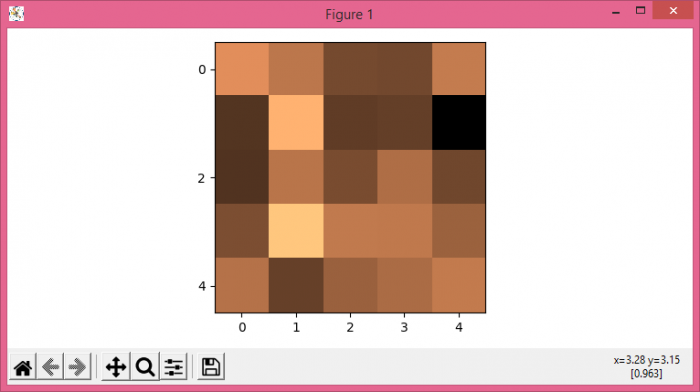# How to plot an image with non-linear Y-axis with Matplotlib using imshow?

To plot an image with non-linear Y-axis with matplotlib using imshow() method, we can take the following steps −

• Set the figure size and adjust the padding between and around the subplots.
• Add a subplot to the current figure.
• Set nonlinear Y-axis ticks.
• Create random data points using numpy.
• Display data as an image, i.e., on a 2D regular raster, with data.
• To display the figure, use show() method.

## Example

import matplotlib.pyplot as plt
import numpy as np

plt.rcParams["figure.figsize"] = [7.50, 3.50]
plt.rcParams["figure.autolayout"] = True

ax = plt.subplot(111)
ax.yaxis.set_ticks([0, 2, 4, 8])

data = np.random.randn(5, 5)

plt.imshow(data, cmap='copper')

plt.show()

## Output